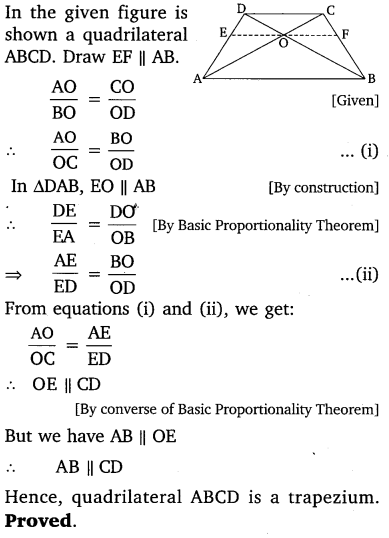# CLASS 10 MATH NCERT SOLUTIONS FOR CHAPTER – 6 TRIANGLES EX – 6.2

## Triangles

Question 1.
In the given figure (i) and (ii), DE || BC. Find EC in (i) and AD in (ii).

Solution: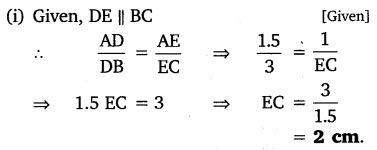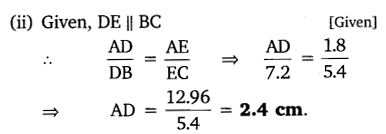Question 2.
E and F are points on the sides PQ and PR respectively of a ∆PQR. For each of the following cases, state whether EF || QR:

(i) PE = 3.9 cm, EQ = 3 cm, PF = 3.6 cm and FR = 2.4 cm
(ii) PE = 4 cm, QE = 4.5 cm, PF = 8 cm and RF = 9 cm
(iii) PQ = 1.28 cm, PR = 2.56 cm, PE = 0.18 cm and PF = 0.36 cm

Solution: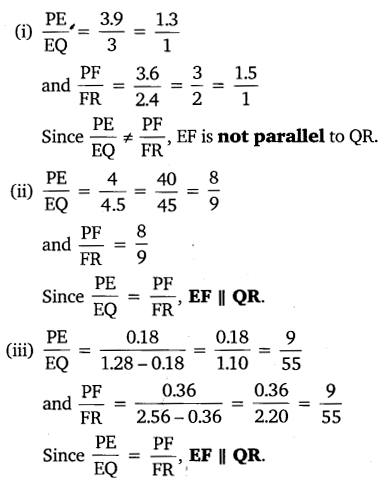Question 3.

In the given figure, if LM || CB and LN || CD.
Prove that$\frac { AM }{ AB } =\frac { AN }{ A{ D }^{ \bullet } }$

Solution: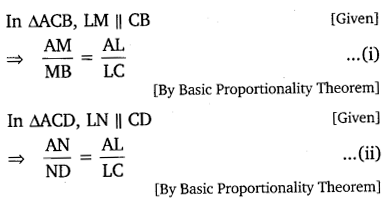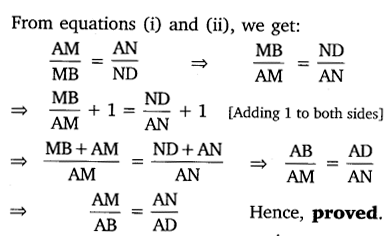Question 4.
In the given figure, DE || AC and DF || AE.
Prove that$\frac { BF }{ FE } =\frac { BE }{ E{ C }^{ \bullet } }$

Solution:Question 5.
In the given figure, DE || OQ and DF || OR. Show that EF || QR.

Solution: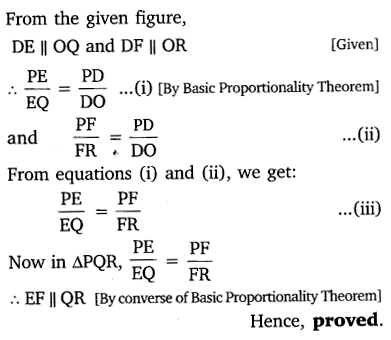Question 6.
In the given figure, A, B, and C are points on OP, OQ and OR respectively such that AB || PQ and AC || PR. Show that BC || QR.

Solution: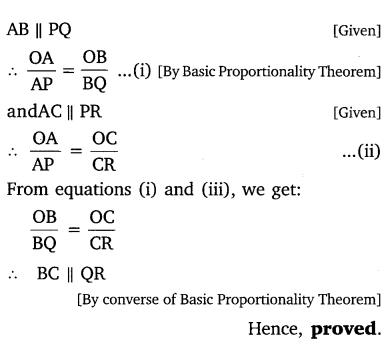Question 7.
Using B.P.T., prove that a line drawn through the mid-point of one side of a triangle parallel to another side bisects the third side. (Recall that you have proved it in class IX)

Solution:
The given figure shows an  ∆ABC in which D is the mid-point of AB.Question 8.
Using converse of B.P.T., prove that the line joining the mid-points of any two sides of a triangle is parallel to the third side.  (Recall that your have done it in class IX)

Solution:
The given figure shows a ΔABC in which D and E are mid-points of sides AB and AC respectively.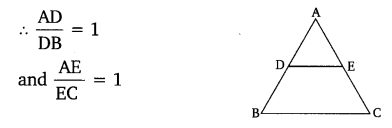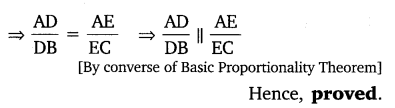Question 9.
ABCD is a trapezium in which AB || DC and its diagonals intersect each other at the point O. Show that

Solution: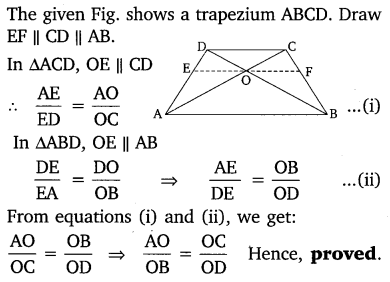Question 10.
The diagonals of a quadrilateral ABCD intersect each other at the point O such that  Show that ABCD is a trapezium.

Solution: